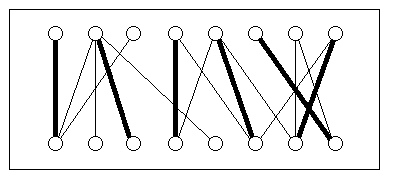Department of Computer Science at the Technische Universität München Chair for Efficient Algorithms Postal address: 80290 München; Premises: Arcisstr.21, 80333 München# Core practical: Design of Algorithms (WS 97/98)

 Lecturer: Prof. Dr. Ernst W. Mayr Time: 6 SWS, Lecture Tue 10:15 - 12:00 Room: Lecture in 1100, Programming exercises can be solved in computer room S2221 Area: Computer Science I (old regulations) Computer Science III (new regulations) (Details here) Prerequisites: Vordiplom; course Effiziente Algorithmen I ( EA II recommended) Coordinator: Thomas Erlebach Registration: the centralized registration takes place at the HP-Halle Certificate: satisfactory solutions to all programming exercises are required for a certificate

## Background

For many problems that are encountered in practice can be solved efficiently if they are modeled as graph problems. For several decades researchers in mathematics and computer science have devised algorithms for the efficient solution of typical graph problems. It has been shown that many problems can be solved by very fast algorithms even though a straightforward implementation requires exponential running-times.Maximum matching in a bipartite graph

So-called matching algorithms, for example, can be used to solve a variety of assignment problems. One such problem could be to assign lecturers to courses in a way that ensures that the maximum possible number of courses can be taught. If every lecturer teaches at most one course and every course is taught at most once, the problem is equivalent to the matching problem in a bipartite graph as depicted in the figure above. The top row of nodes represents lecturers, the bottom row represents courses. An edge between a lecturer node and a course node means that the lecturer is competent to teach the respective course. Now, a valid assignment of courses to lecturers corresponds to a matching in the graph. In the example above, at most six out of eight courses can be taught. The bold edges correspond to such an assignment. The algorithm by Hopcroft and Karp computes maximum matchings in bipartite graphs in time O(sqrt(n)*m), where n is the number of nodes and m is the number of edges of the graph.

Graph algorithms are interesting not only because of their usefulness for the solution of practical problems, but also because of the algorithmic techniques and the used data structures. Therefore, students who intend to take part in this practical course should be prepared to deal with complicated algorithms.

## Content

In the run of this practical course students have to implement different graph algorithms efficiently. In addition, these algorithms should be visualized on the screen using the tool xanimate. Here are some examples for exercises to be solved:depth-first-search, breadth-fist-searchconnected componentsminimum spanning treesshortest pathsmatchingsnetwork flowvertex-coloring planar graphs with 5 colorsapproximating the traveling salesman problem
Most of these algorithms are presented in the courses "Efficient Algorithms and Data Structures I/II". It is recommended to attend these lectures before taking part in this practical course, but it is not required. The goal of the practical course is to help students gain a better understanding of how sophisticated graph algorithms work and to convince them of the benefit obtained by using high-level data structures.

## Structure

The students are given problem sets consisting of programming exercises which have to be solved within one or two weeks. Programs are expected to be written in C++ using LEDA (Library of Efficient Data Types and Algorithms) and xanimate.

LEDA is a class library supplying frequently used data structures such as stacks, queues, priority queues, lists and graphs. Using LEDA reduces substantially the programming effort necessary to implement sophisticated algorithms in C++ efficiently.

xanimate is a tool that consists of a graph editor and a visualization interface. Graph algorithms can determine dynamically how xanimate displays edges and nodes of a graph, enabling the user to observe the progress of the algorithm.

The programming exercises can either be solved in our computer room (S2221), on the workstations in the HP-Halle, or on any PC running Linux (the latter case requires to install xanimate and LEDA first). Groups of two students solve the programming exercises together.

Students and coordinators meet once per week for a two-hour session in which the coordinators discuss solutions to previous exercises and present new exercises and the related theoretical background. In addition, problems encountered by the students can be discussed and questions can be answered by the coordinators.

Students who solve all programming exercises in a satisfactory way receive an ungraded course certificate. If the number of participants is large so that students cooperate in groups, however, an oral examination will have to be passed in addition.

IMPORTANT: The practical "Design of Algorithms" belongs to Computer Science I according to old regulations, and to Computer Science III according to new regulations (valid from November 1996). Students who must take their final exams (DHP) according to new regulations can NOT use the course certificate for Computer Science I. Students who have the possibility to choose between old and new regulations can use the course certificate for either Computer Science I or Computer Science III. Click here for details (in German)!

## Literature:

The following books contain descriptions of algorithms which are the topic of programming exercises in the practical:Turau. Algorithmische Graphentheorie. Addison Wesley, 1996Gibbons. Algorithmic Graph Theory. Cambridge University Press, 1985Mehlhorn. Data Structures and algorithms 2: Graph algorithms and NP-Completeness, Springer-Verlag, 1984Papadimitriou, Steiglitz. Combinatorial Optimization: Algorithms and complexity. Prentice-Hall, 1982Sedgewick. Algorithms. Addison-Wesley, 1988Tarjan. Data Structures and Network Algorithms. SIAM, 1983Reingold, Nievergelt, Deo. Combinatorial Algorithms. Prentice-Hall, 1977

Thomas Erlebach, 1995-10-25, 1996-01-12, 1996-08-01
Tobias Knopff, 1997-04-08